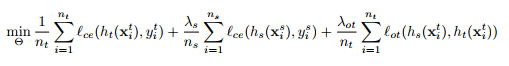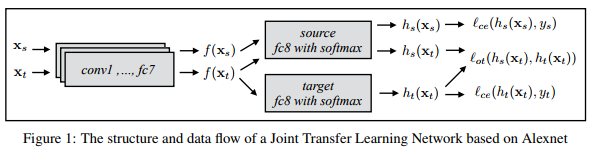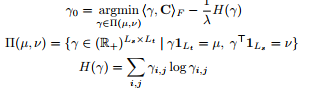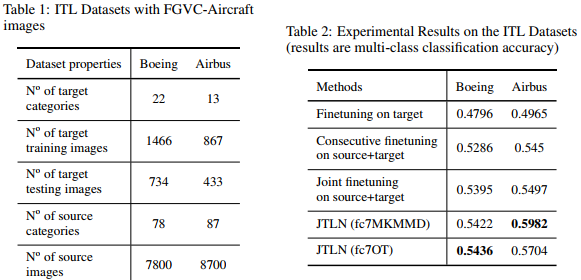## Intro

In this paper, they propose a novel method to jointly fine-tune a Deep Neural Network with source data and target data. By adding an Optimal Transport loss (OT loss) between source and target classifier predictions as a constraint on the source classifier, the proposed Joint Transfer Learning Network (JTLN) can effectively learn useful knowledge for target classification from source data.

## The main idea

Given a small target dataset and a large source dataset, they propose to minimize a combination of three losses:where $$l_{ce}$$ stands for cross-entropy loss and $$l_{OT}$$ for optimal transfer loss. This is illustrated in Figure 1.They implemented the usual regularized optimal transfer losswhere $$H(\gamma)$$ is the entropy of $$\gamma$$ and $$\frac{1}{\lambda}$$ is the regularization weight.

## Results

They report good results for the tranfer between two aircraft image datasets.## Optimal transportation

For a relatively gentle introduction to optimal transportation, please refer to the following tutorial.